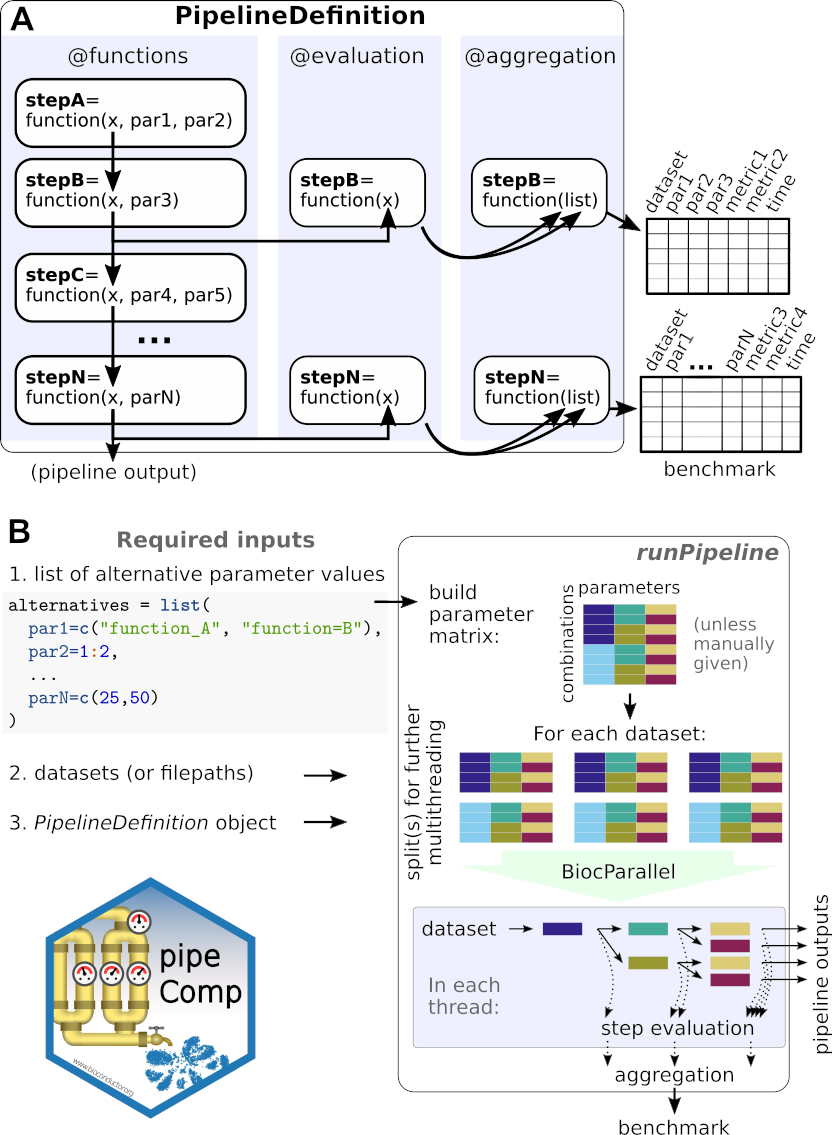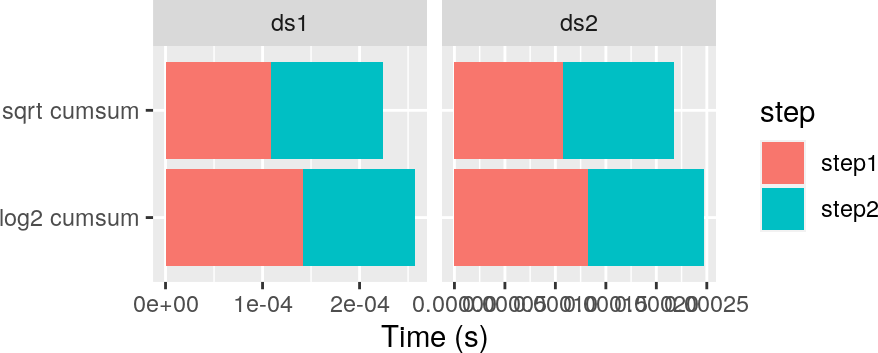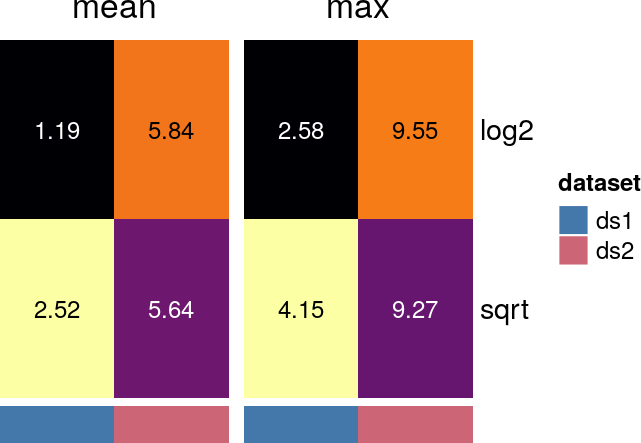# 1 Introduction

pipeComp is a simple framework to facilitate the comparison of pipelines involving various steps and parameters. It was initially developed to benchmark single-cell RNA sequencing (scRNAseq) pipelines:

pipeComp, a general framework for the evaluation of computational pipelines, reveals performant single-cell RNA-seq preprocessing tools
Pierre-Luc Germain, Anthony Sonrel & Mark D Robinson, Genome Biology 2020, doi: 10.1186/s13059-020-02136-7.

However the framework can be applied to any other context. This vignette introduces the package and framework; for information specifically about the scRNAseq pipeline and evaluation metrics, see the pipeComp_scRNA vignette. For a completely different example, with walkthrough the creating of a new PipelineDefinition, see the pipeComp_dea vignette.

# 2 Installation

Install using:

BiocManager::install("plger/pipeComp")

Because pipeComp was meant as a general pipeline benchmarking framework, we have tried to restrict the package’s dependencies to a minimum. To use the scRNA-seq pipeline and wrappers, however, requires further packages to be installed. To check whether these dependencies are met for a given PipelineDefinition and set of alternatives, see ?checkPipelinePackages.

# 3pipeComp overviewFigure 1: Overview of PipeComp and the PipelineDefinition

pipeComp is built around the S4 class PipelineDefinition which defines a basic abstract workflow with different steps (which can have any number of parameters, including subroutines), as depicted in Figure 1A. When a pipeline is executed, each step is consecutively applied to a starting object. Importantly, the step functions are not related to specific methods, but should be generic, whereas the methods to be compared are passed as parameters (e.g. as the name of wrapper functions defined separately from the pipeline). Often, the step function will simply apply the wrapper to the object and do no more. In addition, each step can optionally have evaluation functions, which take the output of the step as an input, and outputs evaluation metrics. Finally, functions can be specified to aggregate these metrics across multiple datasets (in case the evalation output isn’t a data.frame or list of data.frames).

To run a benchmark one in addition needs a set of alternative values to the parameters. For example, alternative values for the parameters depicted in Figure 1 could be defined as follows:

library(pipeComp)
alternatives <- list(
par1=c("function_A", "function_B"),
par2=1:3,
par3=TRUE,
# ...
parN=c(10,25,50)
)

Each parameter (slot of the list) can take any number of alternative scalar values (e.g. character, numeric, logical). The name of functions, like function_A and function_B (which should be loaded in the environment), can be passed if the PipelineDefinition allows it. This means that wrappers should be created for distinct methods, and the name of the wrapper functions passed as alternative parameter values.

Given a PipelineDefinition, a list of alternative parameters and a list benchmark datasets, the runPipeline (Figure 1B) function proceeds through all combinations arguments, avoiding recomputing the same step (with the same parameters) twice and compiling evaluations on the fly to avoid storing potentially large intermediate data:

res <- runPipeline(datasets, alternatives, pipelineDef=PipelineDefinition)

Aggregated evaluation metrics for each combination of parameters, along with computing times, are returned as s with the following structure:

• res$evaluation (step evaluations) •$step1
• $step2 •$metric_table_1
• $metric_table_2 • res$elapsed (running times)
• $stepwise •$step1
• $step2 •$total

In addition to the (aggregated) output returned by the function, runPipeline will produce at least one RDS file (saved according to the output.prefix argument) per dataset: the *.evaluation.rds contain the (non-aggregated) evaluation results at each step, while the .endOutputs.rds files (assuming saveEndResults=TRUE) contain the final output of each combination (i.e. the output of the final step).

The pipeComp package includes a PipelineDefinition for single-cell RNA sequencing (scRNAseq) data. For more information about this application and examples of real outputs, see the pipeComp_scRNA vignette.

## 3.1 Running only a subset of the combinations

Rather than running all possible combinations of parameters, one can run only a subset of them through the comb parameter of runPipeline. The parameter accepts either a matrix (of argument indices) or data.frame (of factors) which can be built manually, but the simplest way is to first create all combinations, and then get rid of the undesired ones:

comb <- buildCombMatrix(alternatives)
head(comb)
##         par1 par2 par3 parN
## 1 function_A    1 TRUE   10
## 2 function_A    1 TRUE   25
## 3 function_A    1 TRUE   50
## 4 function_A    2 TRUE   10
## 5 function_A    2 TRUE   25
## 6 function_A    2 TRUE   50

And then we could remove some combinations before passing the argument to runPipeline:

comb <- comb[ (comb$par1 != "function_A" | comb$par2 == 2) ,]
res <- runPipeline( datasets, alternatives, pipelineDef=PipelineDefinition,
nthreads=3, comb=comb )

## 3.2 Dealing with the PipelineDefinition object

### 3.2.1 Creating a PipelineDefinition

The PipelineDefinition object is, minimally, a set of functions consecutively executed on the output of the previous one, and optionally accompanied by evaluation and aggregation functions. A simple pipeline can be constructed as follows:

my_pip <- PipelineDefinition( list(
step1=function(x, param1){
# do something with x and param1
x
},
step2=function(x, method1, param2){
get(method1)(x, param2) # apply method1 to x, with param2
},
step3=function(x, param3){
x <- some_fancy_function(x, param3)
# the functions can also output evaluation through the intermediate_return slot:
e <- my_evaluation_function(x)
list( x=x, intermediate_return=e)
}
))
my_pip
## A PipelineDefinition object with the following steps:
##   - step1(x, param1)
##   - step2(x, method1, param2)
##   - step3(x, param3)

The PipelineDefinition can also include descriptions of each step or evaluation and aggregation functions. For example:

my_pip <- PipelineDefinition(
list( step1=function(x, meth1){ get(meth1)(x) },
step2=function(x, meth2){ get(meth2)(x) } ),
evaluation=list( step2=function(x) c(mean=mean(x), max=max(x)) ),
description=list( step1="This steps applies meth1 to x.",
step2="This steps applies meth2 to x.")
)
my_pip
## A PipelineDefinition object with the following steps:
##   - step1(x, meth1)
## This steps applies meth1 to x.
##   - step2(x, meth2) *
## This steps applies meth2 to x.

Running it with dummy data and functions:

datasets <- list( ds1=1:3, ds2=c(5,10,15))
alternatives <- list(meth1=c("log","sqrt"), meth2="cumsum")
tmpdir1 <- paste0(tempdir(),"/")
res <- runPipeline(datasets, alternatives, my_pip, output.prefix=tmpdir1)
## Running 2 pipeline settings on 2 datasets using 1 threads
##
##                 Finished running on all datasets
##
##                 Aggregating results...
## Aggregating evaluation results for: step2
res$evaluation$step2
##   dataset meth1  meth2      mean      max
## 1     ds1   log cumsum 0.8283022 1.791759
## 2     ds1  sqrt cumsum 2.5201593 4.146264
## 3     ds2   log cumsum 4.0471780 6.620073
## 4     ds2  sqrt cumsum 5.6352475 9.271329

Computing times can be accessed through res$elapsed; they can either be accessed as the pipeline total for each combination, or in a step-wise fashion. They can also be plotted using plotElapsed (see below). Evaluation results can be accessed through res$evaluation and plotted with evalHeatmap (see below).

### 3.2.2 Manipulating a PipelineDefinition

A number of generic methods are implemented for PipelineDefinition objects, including show, names, length, [, as.list. This means that, for instance, a step can be removed from a pipeline in the following way:

my_pip[-1]
## A PipelineDefinition object with the following steps:
##   - step2(x, meth2) *
## This steps applies meth2 to x.

Steps can be added using the addPipelineStep function:

pip2 <- addPipelineStep(my_pip, name="newstep", after="step1")
pip2
## A PipelineDefinition object with the following steps:
##   - step1(x, meth1)
## This steps applies meth1 to x.
##   - newstep(x)
##   - step2(x, meth2) *
## This steps applies meth2 to x.

Functions for the new step can be specified through the slots argument of addPipelineStep or afterwards through stepFn:

stepFn(pip2, "newstep", type="function") <- function(x, newparam){
do_something(x, newparam)
}
pip2
## A PipelineDefinition object with the following steps:
##   - step1(x, meth1)
## This steps applies meth1 to x.
##   - newstep(x, newparam)
##   - step2(x, meth2) *
## This steps applies meth2 to x.

Finally, the arguments() method can be used to extract the arguments for each step, and the defaultArguments methods can be used to get or set the default arguments.

## 3.3 Merging results of different runPipeline calls

The previous call to runPipeline produced one evaluation file for each dataset:

list.files(tmpdir1, pattern="evaluation\\.rds")
##  "res.ds1.evaluation.rds" "res.ds2.evaluation.rds"

Their aggregation could be repeated manually by reading those files and calling aggregatePipelineResults:

ds <- list.files(tmpdir1, pattern="evaluation\\.rds", full.names = TRUE)
names(ds) <- basename(ds)
res <- aggregatePipelineResults(res1)
## Aggregating evaluation results for: step2

In addition, the results of different calls to runPipeline (with partially different datasets and/or parameter combinations) can be merged together, provided that the PipelineDefinition is the same. To illustrate this, we first make another runPipeline call using slightly different alternative parameter values:

alternatives <- list(meth1=c("log2","sqrt"), meth2="cumsum")
tmpdir2 <- paste0(tempdir(),"/")
res <- runPipeline(datasets, alternatives, my_pip, output.prefix=tmpdir2)
## Running 2 pipeline settings on 2 datasets using 1 threads
##
##                 Finished running on all datasets
##
##                 Aggregating results...
## Aggregating evaluation results for: step2

We then load the (non-aggregated) results of each run from the files, and merge them using mergePipelineResults:

res1 <- readPipelineResults(tmpdir1)
res <- mergePipelineResults(res1,res2)
## $res1 ## ds1 ds2 ## 2 2 ## ##$res2
## ds1 ds2
##   2   2
##
## $merged ## ds1 ds2 ## 2 2 We can then aggregate the results as we do for a single run: res <- aggregatePipelineResults(res) ## Aggregating evaluation results for: step2 res$evaluation$step2 ## dataset meth1 meth2 mean max ## 1 ds1 log2 cumsum 1.194988 2.584963 ## 2 ds1 sqrt cumsum 2.520159 4.146264 ## 3 ds2 log2 cumsum 5.838844 9.550747 ## 4 ds2 sqrt cumsum 5.635248 9.271329 ## 3.4 Handling errors ### 3.4.1 Debugging Errors typically come from the method wrappers, and debugging them from within runPipeline can be complicated by multithreading. For this reason runPipeline wraps additional information around error messages. This would be an example of the error report: Error in dataset mydataset with parameters: filt=lenient, norm=norm.test, k=20 in step normalization, evaluating command: pipDef[["normalization"]](x = x, norm = "norm.test") Error: Error in get(norm)(x): not enough cells! The offending dataset, pipeline step and exact set of parameters is reported, enabling you to try to repeat the error outside, where it is easier to debug. ### 3.4.2 Skipping errors and fixing them afterwards A useful behavior is for runPipeline to continue when encountering errors, so that successful results are not lost. This behavior can be enabled with the skipErrors=TRUE argument. We can illustrate this with our above dummy pipeline, by adding an alternative that is bound to give rise to an error: fragile.fun <- function(x){ if(any(x>10)) stop("Too big!") 2^x } alternatives$meth1 <- c(alternatives$meth1, "fragile.fun") res <- runPipeline(datasets, alternatives, my_pip, output.prefix=tmpdir1, skipErrors=TRUE) ## Running 3 pipeline settings on 2 datasets using 1 threads ## Error in dataset ds2 with parameters: ## meth1=fragile.fun, meth2=cumsum ## in step step1, evaluating command: ## pipDef[["step1"]](x = x, meth1 = "fragile.fun") ## Error: ## Error in get(meth1)(x): Too big! ## ## Finished running on all datasets ## ## Some errors were encountered during the run: ## dataset step ## 1 ds2 meth1=fragile.fun ## All results were saved, but only those of analyses successful across all datasets will be retained for aggregation. ## ## Aggregating results... ## Aggregating evaluation results for: step2 As indicated in the error message, only the analyses successful across all datasets were aggregated: res$evaluation\$step2
##   dataset meth1  meth2     mean      max
## 1     ds1  log2 cumsum 1.194988 2.584963
## 2     ds1  sqrt cumsum 2.520159 4.146264
## 3     ds2  log2 cumsum 5.838844 9.550747
## 4     ds2  sqrt cumsum 5.635248 9.271329

However, all successful results (e.g. including ‘fragile.fun’ on ‘ds1’) were saved in the non-aggregated files. This means that one can then correct the problematic function, re-run only the analyses that failed (i.e. only on ‘ds2’ in this case), and merge the results with the first batch (see ?mergePipelineResults).

## 3.5 Plotting results

pipeComp has two general plotting functions which can be used with any PipelineDefinition: evalHeatmap and plotElapsed. In addition, the package includes pipeline-specific plotting functions (see the pipeComp_scRNA and pipeComp_dea vignettes).

### 3.5.1 Running times

Running times can be plotted with the plotElapsed function:

plotElapsed(res, agg.by=FALSE)In this case we show all tested combinations, but in most cases the agg.by argument will be used to aggregate the results by parameters of interest.

Evaluation results can be plotted through the evalHeatmap function:

evalHeatmap(res, what=c("mean", "max"))By default, the heatmap prints the actual metric values, while the colors is mapped to scaled data. Specifically, pipeComp map colors to the signed square-root of the number of (matrix-wise) Median Absolute Deviations from the (column-wise) median. We found this mapping to be particularly useful because differences in color have comparable meaning across datasets, and the color-scale is not primarily capturing outliers or baseline differences between datasets. This behavior can be changed with the scale parameter of the function. For more complex examples of using evalHeatmap, see its usage in the pipeComp_scRNA vignette, or refer to the function’s help.

For a more complex, ‘real-life’ example of the creation of a PipelineDefinition, see the pipeComp_dea vignette. For a complex example of evaluation outputs, see the pipeComp_scRNA vignette.